# Change Layer and Create New Layer If Needed LISP

## Recommended PostsHello!

So I've been Googling around the internet and am trying to find a LISP that, upon selecting an object, would place that object on a demo layer and if that layer is not present, would create it.

For example,

If I have a point on the V-NODE-CONC layer, upon selecting it, the LISP would switch it to the "V-NODE-CONC-DEMO" layer. If that layer hasn't yet been created, it would copy the "V-NODE-CONC" LAYER, set it to color 240, and add the "-DEMO" suffix.

Thoughts?

Thanks!

##### Share on other sitesTry the following:

```(defun c:demo ( / e i l n s x )
(if (setq s (ssget "_:L" '((8 . "~*-DEMO"))))
(repeat (setq i (sslength s))
(setq x (entget (ssname s (setq i (1- i))))
l (assoc 8 x)
n (strcat (cdr l) "-DEMO")
)
(if (not (tblsearch "layer" n))
(progn
(setq e (entget (tblobjname "layer" (cdr l))))
(entmake (subst (cons 2 n) (assoc 2 e) (subst '(62 . 240) (assoc 62 e) e)))
)
)
(entmod (subst (cons 8 n) l x))
)
)
(princ)
)```

##### Share on other sitesTry the following:

```(defun c:demo ( / e i l n s x )
(if (setq s (ssget "_:L" '((8 . "~*-DEMO"))))
(repeat (setq i (sslength s))
(setq x (entget (ssname s (setq i (1- i))))
l (assoc 8 x)
n (strcat (cdr l) "-DEMO")
)
(if (not (tblsearch "layer" n))
(progn
(setq e (entget (tblobjname "layer" (cdr l))))
(entmake (subst (cons 2 n) (assoc 2 e) (subst '(62 . 240) (assoc 62 e) e)))
)
)
(entmod (subst (cons 8 n) l x))
)
)
(princ)
)```

Fantastic!

One important thing I forgot to mention, is that I need it to work on Civil 3D points and survey figures when we are demoing a topo drawing.

##### Share on other sitesFantastic!

One important thing I forgot to mention, is that I need it to work on Civil 3D points and survey figures when we are demoing a topo drawing.

I don't use Civil 3D (or any of the Verticals), but maybe the ActiveX route will enable compatibility with such objects:

```(defun c:demo ( / e i l n s x )
(if (setq s (ssget "_:L" '((8 . "~*-DEMO"))))
(repeat (setq i (sslength s))
(setq x (vlax-ename->vla-object (ssname s (setq i (1- i))))
l (vla-get-layer x)
n (strcat l "-DEMO")
)
(if (or (tblsearch "layer" n)
(and (setq e (entget (tblobjname "layer" l)))
(entmake (subst (cons 2 n) (assoc 2 e) (subst '(62 . 240) (assoc 62 e) e)))
)
)
(vla-put-layer x n)
)
)
)
(princ)
)

##### Share on other sitesI don't use Civil 3D (or any of the Verticals), but maybe the ActiveX route will enable compatibility with such objects:
```(defun c:demo ( / e i l n s x )
(if (setq s (ssget "_:L" '((8 . "~*-DEMO"))))
(repeat (setq i (sslength s))
(setq x (vlax-ename->vla-object (ssname s (setq i (1- i))))
l (vla-get-layer x)
n (strcat l "-DEMO")
)
(if (or (tblsearch "layer" n)
(and (setq e (entget (tblobjname "layer" l)))
(entmake (subst (cons 2 n) (assoc 2 e) (subst '(62 . 240) (assoc 62 e) e)))
)
)
(vla-put-layer x n)
)
)
)
(princ)
)

It worked for some of the survey figures, but for points and the other survey figures (don't know how there is a difference) I get this..

Thanks for the effort Lee Mac! You're the man!

##### Share on other sitesInteresting - try the following instead:

```(defun c:demo ( / i l n s x )
(if (setq s (ssget "_:L" '((8 . "~*-DEMO"))))
(repeat (setq i (sslength s))
(setq x (vlax-ename->vla-object (ssname s (setq i (1- i))))
l (vla-get-layer x)
n (strcat l "-DEMO")
)
(if (checkmakelayer l n) (vla-put-layer x n))
)
)
(princ)
)
(defun checkmakelayer ( a b )
(cond
(   (tblsearch "layer" b))
(   (setq a (tblobjname "layer" a))
(setq a (entget a))
(entmake
(subst (cons 2 b) (assoc 2 a)
(subst '(62 . 240) (assoc 62 a)
(vl-remove-if '(lambda ( x ) (or (= 'ename (type (cdr x))) (= 102 (car x)))) a)
)
)
)
)
)
)

I'm guessing the layer could have an extension dictionary present which may be causing problems when duplicating it.

##### Share on other sitesInteresting - try the following instead:
```(defun c:demo ( / i l n s x )
(if (setq s (ssget "_:L" '((8 . "~*-DEMO"))))
(repeat (setq i (sslength s))
(setq x (vlax-ename->vla-object (ssname s (setq i (1- i))))
l (vla-get-layer x)
n (strcat l "-DEMO")
)
(if (checkmakelayer l n) (vla-put-layer x n))
)
)
(princ)
)
(defun checkmakelayer ( a b )
(cond
(   (tblsearch "layer" b))
(   (setq a (tblobjname "layer" a))
(setq a (entget a))
(entmake
(subst (cons 2 b) (assoc 2 a)
(subst '(62 . 240) (assoc 62 a)
(vl-remove-if '(lambda ( x ) (or (= 'ename (type (cdr x))) (= 102 (car x)))) a)
)
)
)
)
)
)

I'm guessing the layer could have an extension dictionary present which may be causing problems when duplicating it.

HO-LY SHET!! YES!!

I'm going to talk to my boss about kicking down a donation for you.

Thanks Lee Mac!!

##### Share on other sites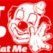HO-LY SHET!! YES!!

I'm going to talk to my boss about kicking down a donation for you.

Thanks Lee Mac!!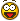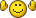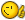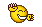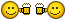##### Share on other sitesHO-LY SHET!! YES!!

I'm going to talk to my boss about kicking down a donation for you.

Thanks Lee Mac!!

Many thanks Paul! - I'm pleased that the code is working well.

##### Share on other sitesAttempting to swap out the suffix of -demo for a prefix sd-; also attempting to maintain the original layers color. However the lisp does nothing.

I have cobbled together the following code:

```(defun c:demo ( / i l n s x )
(if (setq s (ssget "_:L" '((8 . "SD*~"))))
(repeat (setq i (sslength s))
(setq x (vlax-ename->vla-object (ssname s (setq i (1- i))))
l (vla-get-layer x)
n (strcat l "SD-")
)
(if (checkmakelayer l n) (vla-put-layer x n))
)
)
(princ)
)
(defun checkmakelayer ( a b )
(cond
(   (tblsearch "layer" b))
(   (setq a (tblobjname "layer" a))
(setq a (entget a))
(entmake
(subst (cons 2 b) (assoc 2 a)
(subst '(62 . 256) (assoc 62 a)
(vl-remove-if '(lambda ( x ) (or (= 'ename (type (cdr x))) (= 102 (car x)))) a)
)
)
)
)
)
)

##### Share on other sitesTry the following:

```(defun c:demo ( / i l n s x )
(if (setq s (ssget "_:L" '((8 . "~SD-*"))))
(repeat (setq i (sslength s))
(setq x (vlax-ename->vla-object (ssname s (setq i (1- i))))
l (vla-get-layer x)
n (strcat "SD-" l)
)
(if (checkmakelayer l n) (vla-put-layer x n))
)
)
(princ)
)
(defun checkmakelayer ( a b )
(cond
(   (tblsearch "layer" b))
(   (setq a (tblobjname "layer" a))
(setq a (entget a))
(entmake
(subst (cons 2 b) (assoc 2 a)
(vl-remove-if '(lambda ( x ) (or (= 'ename (type (cdr x))) (= 102 (car x)))) a)
)
)
)
)
)

##### Share on other sitesMany thanks Dr. Lee

##### Share on other sitesInteresting - try the following instead:
```(defun c:demo ( / i l n s x )
(if (setq s (ssget "_:L" '((8 . "~*-DEMO"))))
(repeat (setq i (sslength s))
(setq x (vlax-ename->vla-object (ssname s (setq i (1- i))))
l (vla-get-layer x)
n (strcat l "-DEMO")
)
(if (checkmakelayer l n) (vla-put-layer x n))
)
)
(princ)
)
(defun checkmakelayer ( a b )
(cond
(   (tblsearch "layer" b))
(   (setq a (tblobjname "layer" a))
(setq a (entget a))
(entmake
(subst (cons 2 b) (assoc 2 a)
(subst '(62 . 240) (assoc 62 a)
(vl-remove-if '(lambda ( x ) (or (= 'ename (type (cdr x))) (= 102 (car x)))) a)
)
)
)
)
)
)

I'm guessing the layer could have an extension dictionary present which may be causing problems when duplicating it.

Hi guys. First post here. I would like to express my admiration to all contributors here. Plenty of usable info posted.

This code works great and I am trying to add some to it. Is it possible to assign the color of the new layer based on the color of the original layer:

If the original layer color is 1 the new layer to be 201; if it is 2 to become 202, etc. for colors from 1 to 8. I assume a loop or condition check has to be added to (subst '(62 . 240) (assoc 62 a) but programming is not my strength.

Thanks

##### Share on other sitesWelcome to CADTutorTry the following quick modification -

```(defun c:demo ( / i l n s x )
(if (setq s (ssget "_:L" '((8 . "~*-DEMO"))))
(repeat (setq i (sslength s))
(setq x (vlax-ename->vla-object (ssname s (setq i (1- i))))
l (vla-get-layer x)
n (strcat l "-DEMO")
)
(if (checkmakelayer l n) (vla-put-layer x n))
)
)
(princ)
)
(defun checkmakelayer ( a b / c )
(cond
(   (tblsearch "layer" b))
(   (setq a (tblobjname "layer" a))
(setq a (entget a)
c (abs (cdr (assoc 62 a)))
)
(entmake
(subst (cons 2 b) (assoc 2 a)
(subst
(cons  62 (if (< c 9) (+ c 200) c))
(assoc 62 a)
(vl-remove-if '(lambda ( x ) (or (= 'ename (type (cdr x))) (= 102 (car x)))) a)
)
)
)
)
)
)

##### Share on other sitesIt works flawlessly. Thank you Lee!

##### Share on other sitesYou're most welcome!

##### Share on other sitesHi,

I'm having similar problem, I have prepared drawing template with many layers defined by specification, something like this:

```first_layer_name
first_layer_name-description1
first_layer_name-description2
first_layer_name-description3
first_layer_name-description4

second_layer_name
second_layer_name-description1
second_layer_name-description2
second_layer_name-description3
second_layer_name-description4

third_layer_name
third_layer_name-description1
third_layer_name-description2
third_layer_name-description3
third_layer_name-description4
...```

Description is always same for all layers (currently there are 4 possible variants, like above).

So my job is to go through drawing, select elements in base layer (without description) and change layer to one with description, depending on situation, sometimes it should go to layer with description1, sometimes in others.

I would like to automate this with keyboard shortcuts, something like this: select an element in "first_layer_name" and press "SHIFT+1" and it would go to layer "first_layer_name-description1", with "SHIFT+2" it should go to "first_layer_name-description2" and so on. Lisp should work in other way too (if I make mistake), select an element in "first_layer_name-description2" press "SHIFT+1" and it would go to "first_layer_name-description1".

I figured out how to create keyboard shortcuts - I created macro which runs lisp command and created Shortcut Key for macro and it works, so only problem left is pretty complicated lisp routine.

## Join the conversation

You can post now and register later. If you have an account, sign in now to post with your account.
Note: Your post will require moderator approval before it will be visible.×   Pasted as rich text.   Paste as plain text instead

Only 75 emoji are allowed.